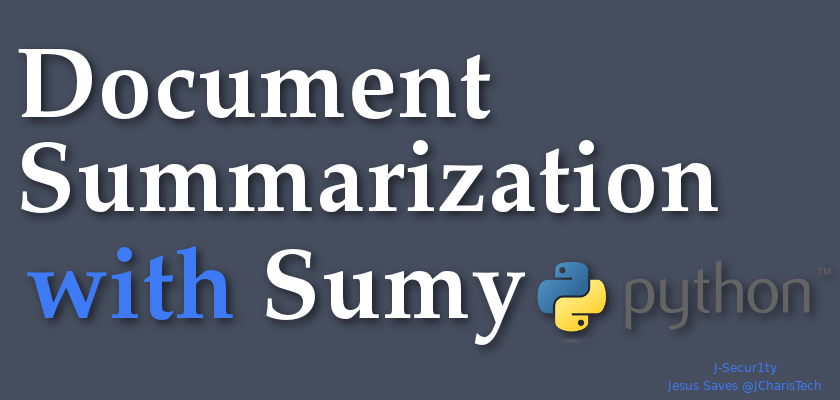# How To Summarize Text or Document With Sumy

### Text Summarization Using Sumy & Python

In this tutorial we will learn about how to summarize documents or text using  a simple yet powerful package called Sumy.

#### How to Installation

• pip install sumy
• Sumy offers several algorithms and methods for summarization such as:
• Luhn – heurestic method
• Latent Semantic Analysis
• Edmundson heurestic method with previous statistic research
• LexRank – Unsupervised approach inspired by algorithms PageRank and HITS
• TextRank
• SumBasic – Method that is often used as a baseline in the literature
• KL-Sum – Method that greedily adds sentences to a summary so long as it decreases the KL Divergence.

Let us see how it works.

In :
```# Load Packages
import sumy
```
In :
```from sumy.parsers.plaintext import PlaintextParser
from sumy.nlp.tokenizers import Tokenizer
from sumy.summarizers.lex_rank import LexRankSummarizer
```
In :
```document1 ="""Machine learning (ML) is the scientific study of algorithms and statistical models that computer systems use to progressively improve their performance on a specific task. Machine learning algorithms build a mathematical model of sample data, known as "training data", in order to make predictions or decisions without being explicitly programmed to perform the task. Machine learning algorithms are used in the applications of email filtering, detection of network intruders, and computer vision, where it is infeasible to develop an algorithm of specific instructions for performing the task. Machine learning is closely related to computational statistics, which focuses on making predictions using computers. The study of mathematical optimization delivers methods, theory and application domains to the field of machine learning. Data mining is a field of study within machine learning, and focuses on exploratory data analysis through unsupervised learning.In its application across business problems, machine learning is also referred to as predictive analytics."""
```
In :
```# For Strings
parser = PlaintextParser.from_string(document1,Tokenizer("english"))
```
In [ ]:
```# For Files
parser = PlaintextParser.from_file(file, Tokenizer("english"))
```

### Using LexRank

• unsupervised approach to text summarization based on graph-based centrality scoring of sentences.
• The main idea is that sentences “recommend” other similar sentences to the reader. Thus, if one sentence is very similar to many others, it will likely be a sentence of great importance
• Standalone pkg pip install lexrank
In :
```# Using LexRank
summarizer = LexRankSummarizer()
#Summarize the document with 2 sentences
summary = summarizer(parser.document, 2)
```
In :
```for sentence in summary:
print(sentence)
```
```Machine learning (ML) is the scientific study of algorithms and statistical models that computer systems use to progressively improve their performance on a specific task.
Machine learning algorithms build a mathematical model of sample data, known as "training data", in order to make predictions or decisions without being explicitly programmed to perform the task.
```

#### Using Luhn

• Based on frequency of most important words
In :
```from sumy.summarizers.luhn import LuhnSummarizer
```
In :
```summarizer_luhn = LuhnSummarizer()
summary_1 =summarizer_luhn(parser.document,2)
```
In :
```for sentence in summary_1:
print(sentence)
```
```Machine learning algorithms build a mathematical model of sample data, known as "training data", in order to make predictions or decisions without being explicitly programmed to perform the task.
Data mining is a field of study within machine learning, and focuses on exploratory data analysis through unsupervised learning.In its application across business problems, machine learning is also referred to as predictive analytics.
```

#### Using LSA

• Based on term frequency techniques with singular value decomposition to summarize texts.
In :
```from sumy.summarizers.lsa import LsaSummarizer
```
In :
```summarizer_lsa = LsaSummarizer()
summary_2 =summarizer_lsa(parser.document,2)
```
In :
```for sentence in summary_2:
print(sentence)
```
```Machine learning (ML) is the scientific study of algorithms and statistical models that computer systems use to progressively improve their performance on a specific task.
Machine learning is closely related to computational statistics, which focuses on making predictions using computers.
```
In :
```## Alternative Method using stopwords
from sumy.nlp.stemmers import Stemmer
from sumy.utils import get_stop_words
summarizer_lsa2 = LsaSummarizer()
summarizer_lsa2 = LsaSummarizer(Stemmer("english"))
summarizer_lsa2.stop_words = get_stop_words("english")
```
In :
```for sentence in summarizer_lsa2(parser.document,2):
print(sentence)
```
```Machine learning (ML) is the scientific study of algorithms and statistical models that computer systems use to progressively improve their performance on a specific task.
The study of mathematical optimization delivers methods, theory and application domains to the field of machine learning.
```
You can also check out the video tutorial here

By Jesse JCharis

Jesus Saves@JCharisTech

### 3 thoughts on “How To Summarize Text or Document With Sumy”

1.Hi – great introduction.

How does one incorporate machine learning in order to “train” the program towards better summarization?

2.Nice post. I was checking constantly this blog and I am impressed! Very useful info specially the last part 🙂 I care for such info much. I was seeking this certain information for a long time. Thank you and best of luck.

3.Good article! We are linking to this particularly great post on our site. Keep up the good writing.